# 2nd PUC Chemistry Question Bank Chapter 6 General Principles and Processes of Isolation of Elements

You can Download Chapter 6 General Principles and Processes of Isolation of Elements Questions and Answers, Notes, 2nd PUC Chemistry Question Bank with Answers Karnataka State Board Solutions help you to revise complete Syllabus and score more marks in your examinations.

## Karnataka 2nd PUC Chemistry Question Bank Chapter 6 General Principles and Processes of Isolation of Elements

### 2nd PUC Chemistry General Principles and Processes of Isolation of Elements NCERT Textbook Questions and Answers

Question 1.
Copper can be extracted by hydrometallurgy but not zinc. Explain.
E° value of Zn2+/Zn = – 0·76V is less than that of Cu2+/Cu = + 0·34 V. This means that zinc is a stronger reducing agent than copper. In solution, Cu2+ ions can be easily displaced by reducing agents like Fe and Zn which don’t react with water.
Fe (s) + Cu2+ (aq) → Fe2+ (aq) + Cu (s)
In order to isolate zinc by hydrometallurgy, we require stronger reducing agents like Ca, Mg, Al, etc. However, all of them react with water to evolve hydrogen gas. Therefore, these cannot be used for this purpose. Thus, zinc cannot be extracted by hydrometallurgy.

Question 2.
What is the role of the depressant in froth floatation process?
In the froth floatation-process, the role of the depressant is to prevent one type of sulphide ore particles from forming the froth with air bubbles. For eg., NaCN is used as a depressant to separate lead sulphide (PbS) ore from zinc sulphide (ZnS) ore. The reason being that NaCN forms a zinc complex, Na2[Zn (CN)4] on the surface of ZnS thereby preventing the formation of the froth. Under these conditions, only PbS forms froth and hence can be separated from ZnS ore.
4 NaCN + ZnS → Na2 [Zn (CN)4] + Na2S
Sod. tetracy emozincate (II)Question 3.
Why is the extraction of copper from pyrites more difficult than that from its oxide ore through reduction?
The standard free energy (∆fG°) of the formation of Cu2 S is more -ve than those of H2S and CS2. Therefore, Cu2S cannot be reduced by carbon or H2. The following two reactions do not occur. ∆rG° for these reactions is positive.
Cu2S + H2 → 2Cu + H2S;
2CU2S + C → 4Cu + CS2
In contrast, ∆fG° of Cu2O is less -ve than that of CO and hence carbon can easily reduce Cu2O to Cu.
Cu2O(s) + C(s) → 2Cu + CO(g)
It is because of this reason that the extraction of copper from pyrite is difficult than from its oxide ore.

Question 4.
Explain (i) Zone refining (ii) Column chromatography.
(i) This method is based on the principle that the impurities are more soluble in the molten state of metal (the melt) than in the solid-state. In the process of zone refining, a circular mobile heater is fixed at one end of a rod of impure metal. As the heater moves the molten zone of the rod also moves with it. As a result, pure metal crystallizes out of the melt and the impurities pass onto the adjacent molten zone. This process is repeated several times, which leads to the segregation of impurities at one end of the rod. Then the end with impurities is cut off. Silicon, boron, gallium, indium, etc can be purified by this process.

(ii) Column chromatography is a technique used to separate components of a mixture where components are in minute quantities. In chromatography, there are two phases: the mobile phase and the stationary phase. The stationary phase is immobile and immiscible. Al2O3 column is usually used as the stationary phase in column chromatography. The mobile phase may be a gas, liquid or supercritical fluid in which the sample extracts dissolve. Then the mobile phase is forced to wave through the stationary phase. The component that is more strongly absorbed on the column takes a long time to travel than the component weakly, absorbed.

Question 5.
Out of C and CO, which is a better reducing agent at 673 K ?
If we look carefully at the Ellingham diagram (Fig. 6.7), we find that at 983 K, the curves intersect. AG° involving a change of CO to CO2 (CO, CO2) is more as compared to the value involving a change of C to CO2 (C, CO2). This means that at this temperature or above it, coke (C) is a better reducing agent. However, below this temperature, the reverse happens. Therefore, carbon monoxide (CO) is a better reducing agent at 673 K than coke (C).

Question 6.
Name the common elements present in the anode mud in electrolytic refining of copper. Why are they so present?
The common elements present in the anode mud are antimony, selenium, tellurium, silver, gold and platinum. These elements, being less reactive, are not affected by CuSO4 – H2SO4 solution and hence settle down under anode as anode mud.Question 7.
Write down the reactions taking place in different zones in the blast furnace during the extraction of iron.
At 500 – 800 K (lower temperature range in the blast furnace)
3 Fe2O3 + CO → 2 Fe3O4 + CO2 (6.28)
Fe3O4 + 4 CO → 3Fe + 4 CO2 (6.29)
Fe2O3 + CO → 2 FeO + CO2 (6.30)
At 99 – 1500 K (higher temperature range in the blast furnace):
C + CO2 → 2 CO (6.31)
FeO + CO → Fe + CO2 (6.32)

Question 8.
Write chemical reactions taking place in the extraction of zinc from zinc blende.
Various steps involve are:
(a) Concentration – The ore is crushed and then concentrated: froth flotation process.
(b) Roasting – The concentrated ore is then roasted in presence of excess air at about 1200k when zinc oxide (ZnO) is formed.(c) Reduction – Zinc oxide obtained above is mixed with powdered coke and heated to 1673K in a fire clay retort when it is reduced to Zinc metal.At 1673 k, zinc metal being volatile (b.p. 1180 K), distills over and is condensed.

(d) Electrolytic refining – Impure zinc is made the anode while cathode consists of a sheet of pure zinc. The electrolyte consists of ZnSO4 solution acidified with dil H2SO4. On passing, current pure Zn gets deposited on the cathode.

Question 9.
State the role of silica in the metallurgy of copper.
During roasting, copper pyrites are converted into a mixture of FeO and Cu2O,To remove FeO (basic) an acidic flux silica is added during smelting. FeO then combines with SiO2 to form ferrous silicate (FeSi03) slag which floats over molten matter.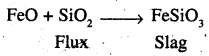Thus, the role of silica in the metallurgy of copper is to remove iron oxide as slag.

Question 10.
What is meant by the term “chromatography”?
The term chromatography means colour writing (Greek: Chroma means colour; graphy means writing). Initially, chromatography was used to identify and separate only the coloured components or constituents. But now, any type of constituents can be separated even if available in a small amount.

Question 11.
What criterion is followed for the selection of the stationary phase in chromatography?
The stationary phase is selected in such a way that the impurities are more strongly adsorbed or are more soluble in the stationary phase than components to be purified. Thus, when the column is extracted, the impurities will be retained by the stationary phase while the pure component is easily eluted.Question 12.
Describe a method for refining nickel.
When impure nickel is heated in a current of CO at 330 – 350K, it forms volatile nickel tetracarbonyl leaving behind the impurities. The nickel tetracarbonyl thus obtained is then heated to a higher temperature (450 – 470K), when it undergoes thermal decomposition to give pure nickel.

Question 13.
How can you separate alumina from silica in a bauxite ore associated with silica? Give equations, if any.
Pure alumina may be separated from the bauxite by Baeyer’s process as discussed below. The I bauxite ore associated with Silica is heated with a concentrated (45%) solution of NaOH at473-523 K and 35- 36 bar pressure. Under these conditions, alumina dissolves as sodium metal aluminate and Silica as sodium silicate leaving behind the impurities.The resulting solution is filtered to remove the dissolved impurities, if any, and neutralized by passing CO2 gas. Thereafter, the solution is seeded with freshly prepared samples of hydrated alumina when hydrated alumina gets precipitated leaving sodium silicate in the solution.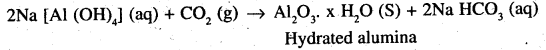The hydrated alumina thus precipitated is filtered, dried and heated to give back pure Al2O3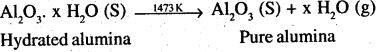Alternatively, Serpeck’s process may be employed for the purification of white bauxite which contains Silica as the impurity.
The ore is powdered, mixed with coke and heated to 2073K in an atmosphere of N2 gas. Alumina combines with N2 from aluminium nitride while Silica is reduced to silicon which volatilizes off at this temperature.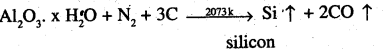Aluminium nitride thus obtained is hydrolysed by water to form aluminium hydroxide which, when ignited, gives pure alumina.This process has one distinct advantage that ammonia is obtained as a by-product.

Question 14.
Giving examples, differentiate between ‘roasting’ and ‘calcination’.
The process of converting carbonates and hydroxide ores of metals to their respective oxides by heating them strongly below their melting points either in the absence or limited supply of air is called calcination.
For example,On the other hand, the process of converting a sulphide ore into its metallic oxide by heating strongly below its melting point in excess of air is called roasting. For example,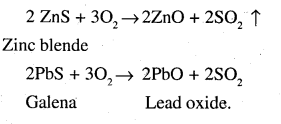Question 15.
How is ‘cast iron’ different from ‘pig iron”?
The iron obtained from blast furnace is called pig iron. It contains about 4% carbon and many other impurities in smaller amount (eg., S, P, Si and Mn). Cast iron is made by melting pig iron with scrap iron and coke using hot air blast. It has slightly lower carbon content (about 3%) and is extremely hard and brittle.

Question 16.
Differentiate between “minerals” and “ores”.
The natural substances in which the metal or their compounds occur in the earth are called minerals. The mineral has a definite composition. It may be a single compound or complex mixture. The minerals from which the metals can be conveniently and economically extracted are known as ores. All the ores arc minerals but all minerals cannot be ores, e.g., both bauxite (Al2O3.xH2O) and clay (Al2O3.2SiO2.2H2O) are minerals of aluminium. It is bauxite which is used for the extraction of aluminium and not clay. Thus bauxite is an ore of aluminium.

Question 17.
Why copper matte is put in a silica-lined converter?
Copper matte chiefly consists of Cu2S along With some unchanged FeS. When a blast of hot air is passed through molten matte taken in a silica lined converter, FeS present is oxidised to FeO which combines with silica (SiO2) to form FeSiO3 slag.When the whole of iron has been removed as slag, some of the Cu2S undergoes oxidation to form Cu20 which then reacts with more Cu2S to form copper metal.
2 Cu2S + 3O, → 2 Cu2O + 2SO2
2Cu2O + Cu2S → 6 Cu + SO2
Thus, copper matte is heated in Silica lined converter to remove FeS present in matte as FeSiO3 slag.

Question 18.
What is the role of cryolite in the metallurgy of aluminium?
In the metallurgy of aluminium, the metal is to be isolated from alumina (Al2O3) by carrying out its electrolytic reduction. The melting point of alumina as such is 2323 K. It is, therefore, mixed with cryolite (Na3AlF6) which lowers its melting point* to 1173 K. Moreover, cryolite also increases the electrical conductivity of alumina which is a poor conductor.

Question 19.
How is leaching carried out in the case of low-grade copper ores?
The leaching of the low grade copper ores is carried out with acids in presence of air when copper goes into solution as Cu2+ ions. Thus
2Cu (s) + 2H2SO4 (aq) + O2 (g) → 2CuSO4 (aq) + 2H2O(l)
or Cu (s) + 2H+ (aq) + $$\frac { 1 }{ 2 }$$ O2 (g) → Cu2+ (aq) + H2O (l).

Question 20.
Why is zinc not extracted from zinc oxide through reduction using CO?
This is because the standard free energy of the formation of CO2 from CO is higher than that of the standard free energy of the formation of ZnO from Zn.

Question 21.
The value of Δf G° for the formation of Cr2 O3 is – 540 kJ mol-1 and that of Al2 O3 is – 827 kJ mol-1. Is the reduction of Cr2 O3 possible with Al?
The two equation are :
$$\frac { 4 }{ 3 }$$ Al (s) + O2 (g) $$\frac { 2 }{ 3 }$$Al2O3 (S); ΔfG° Al,
Al2O3 = -827 kjmol-1 …………..(i)
$$\frac { 4 }{ 3 }$$ Cr (s) + O2 (g) $$\frac { 2 }{ 3 }$$cr2O3 (s); ΔfG° = -827 kjmol-1 ……………(ii)

subtracting (ii) from (i), we have
$$\frac { 4 }{ 3 }$$ Al (s) + $$\frac { 2 }{ 3 }$$ Cr2O2 (s) → $$\frac { 2 }{ 3 }$$Al2O3(S)
+ $$\frac { 4 }{ 3 }$$cr(s): ΔfG° = -287kJmol-1
Since ΔfG° of the combined redox reaction is negative, therefore, reduction of Cr2O3by Al is possible.

Question 22.
Out of C and CO, which is a better reducing agent for ZnO?
The two reduction reactions are:
ZnO(s) + C(s) → Zn(s) + CO(g) …(i)
ZnO(s) + CO(g) → Zn(s) + CO2(g) …(ii)
In the first case, there is an increase in the magnitude of ∆S° while in the second case, it almost remains the same. In other words, AG° will have a more negative value in the first case when C(s) is reducing than in the second case when CO(g) acts as the reducing agent. Therefore, C(s) is a better reducing agent.Question 23.
The choice of a reducing agent in a particular case depends on the thermodynamic factor. How far do you agree with this statement? Support your opinion with two examples.
The thermodynamic factor helps us in choosing a suitable reducing agent for the reduction of a particular metal oxide to metal. The feasibility of thermal reduction can be predicted on the basis of ∆fG° vs T plots for the formation of oxides, known as the Ellingham diagram. From the diagram, it can be predicted that metals for which ∆fG° oxides is more negative can reduce those metal oxides for which the ∆fG°, oxides are less negative. In other words, one will reduce the oxides of other metals which lie above in Ellingham’s diagram, because ∆rG° of the combined redox reaction will be -ve by an amount equal to the difference in ∆fG° of the two metals oxides. For example, Al can reduce Cr2O3 but not MgO. Similarly, C can reduce ZnO to Zn but not CO. Thus, the choice of a particular reducing agent depends upon the thermodynamic factor.

Question 24.
Name the processes from which chlorine is obtained as a by-product. What will happen if an aqueous solution of NaCl is subjected to electrolysis?
Sodium metal is prepared by Down’s process. It involves the electrolysis of a fused mixture of NaCl and CaCl2 at 873K. During electrolysis, sodium is discharged at the cathode while Cl2 is obtained at the anode as a by-product.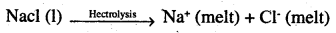At cathode : Na+ (melt) + e → Na (s)
At anode : Cl(melt) → Cl (g) + e
2Cl (g) → Cl2(g)
If, however, an aqueous solution of NaCl is electrolyzed, is evolved at the cathode while Cl2 is obtained at the anode. The reason being that the E° of Na+/ Na redox couple is much lower (∈° = -2.71V) than that of H2O (∈°H2O/H2 = -0.83V) and hence water is reduced to H, in preference to Na+ ions. NaOH is however obtained in the solution.
At anode:Cl (aq) → Cl (aq) + e
2 Cl (g) → Cl2(g)
At cathode: 2H20(1) + 2e → H2 (g) + 2OH (aq)

Question 25
What is the role of a graphite rod in the electrometallurgy of aluminium?
In the electrometallurgy of aluminium, a fused mixture of alumina, cryolite, and fluorspar (CaF2) is electrolysed using graphite as anode and graphite lined iron as a cathode. During electrolysis, Al is liberated at the cathode while CO and CO2 are liberated at the anode.

At cathode Al3+ (melt) → Al (l)
At anode : C (s) + O2- (melt) → CO (g) + 2e
C (s) + 2O2- (melt) → CO2 (g) + 4e

If instead of graphite, some metal is used as the anode, then O2 liberated will not only oxidised the metal of the electrode but would also convert some of the A1 liberated at the cathode back to Al2O3.

Since graphite is much cheaper than any metal, therefore, graphite is used as the anode. Thus the role of graphite in the electrometallurgy of Al is to prevent the liberation of O2 at the anode which may otherwise oxidise some of the liberated Al back to Al2O3.

Question 26.
Outline the principles of refining of metals by following methods :
(i) Zone refining
(ii) Electrolytic refining
(iii) Vapour phase refining.
(i) Zone refining (Fractional Crystallisation): This method is used only if the metal in an almost pure state is required. Metals like germanium and gallium which are used in semiconductors are purified by this method. The principle of zone refining is based on the fact that impurities are more soluble in molten metal than in solid metal.
In other words, we can say that when an impure metal in the molten state is allowed to cool, only the metal crystallises while the impurities remain present in the molten mass or melt.

(ii) Electrolytic refining: This method is commonly used for the purification of the metals like Cu, Ag, Zn, Ni, etc. The impure metal converted into a block which is made the anode in an electrolytic cell The electrolyte is the solution of the soluble salt of the same metal, preferably a double salt. On passing electric current, metal ions from the electrolyte are reduced to the metal which is deposited in the cathode. An equivalent amount of the pure metal from the anode gets oxidised and the metal ions (or cations) go into the solution.

(iii) Vapour phase refining: In this method, the impure metal is converted into a volatile compound, by a suitable method leaving behind the impurities. The volatile compound formed being unstable decomposes at an elevated temperature to give pure metal. A few cases are discussed.

Question 27.
Predict conditions under which A1 might be expected to reduce MgO.
Above 1350°C, the standard Gibbs free energy of formation of Al2O2 from Al is less than that at MgO from Mg. Therefore, above 1350°C, A1 can reduce MgO.

### 2nd PUC Chemistry General Principles and Processes of Isolation of Elements NCERT Additional Questions and Answers

Question 1.
State briefly the principles which serve as the basis for the following operations in metallurgist.
(i) Froth flotation process
(ii) Zone refining ‘
(iii) Refining by liquation (Delhi 2008)
(i) The principle of froth floatation is that the mineral particles are wetted by oils and gangue particles by water.
(ii) Zone refining is based on the principle that the impurities are more soluble in the melt than in the solid-state of the metal
(iii) In refining by liquation method, a low melting metal like a tin can be made to flow on a sloping surface. In this way, it is separated from higher melting impurities.Question 2.
What chemical principle is involved in choosing a reducing agent for getting the metal from its oxide ore? Consider the metal oxides, Al2O3 and Fe2O3, and justify the choice of reducing agent in each case.
(i) Thermodynamic factor helps us in choosing a suitable reducing agent for the reduction of a particular metal oxide to the metallic state.
(ii) Any metal will reduce the oxides of other metals which lie above it in the Ellingham diagram because the standard free energy change (∆r Gθ) of the combined redox reaction will be negative by an amount equal to the difference in ∆r Gθ of two metal oxides. Thus both Al and Zn can reduce Fe2O3 to Fe but cannot reduce Al2O3 to Al.

3 (a) Name two alloys of copper.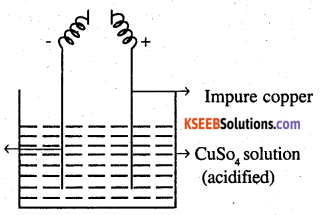From the figure, give the electrode reaction. What is this process called?
(a) Bras: Cu (60%) + Zn (40%)
Bronze: Cu (90%) + Sn (10%)
(b) This process is known as electrolyte refining. The impure metal is used as an anode and pure metal is used as a cathode. The electrolyte is a solution of a suitable salt of the metal. On passing electric current, the pure metal is deposited on the cathode. The solid impurities fall to the bottom of the cell and are recovered as anode mud. The soluble impurities go to the solution. For the purification of copper sulphate and the electrode, reactions are
Anode: Cu → Cu2 + 2e
Cathode: Cu2 + 2e → Cu

Question 4.
The preparation of a metal from its concentrated ore is known as extraction?

1. Explain the principle behind smelting.
2. Explain the extraction of a metal by the reduction of metal oxide using aluminium. Also, explain the practical application of it.
3. Give two examples in which the metal is formed by auto-reduction of the concentrated ore.

1. The extraction of a metal from the metal oxide by reduction with carbon is known as smelting. This method is used for the extraction of Pb, Zn, Fe, Cu and Sn.

2. Metallic oxides of chromium and manganese are reduced by strongly electropositive metals such as aluminium. This process is known as the aluminothermic process.
Cr2O3 + 2Al → 2Cr + Al2O3
3MnO2 + 4Al → 3Mn + 2Al2O3
Since these reactions are highly exothermic and molten metal is produced, it is used in the thermite welding process.

3. When the sulphide ores of Hg, Cu, Pb etc. are heated in air, a part of these sulphide ores is changed into oxide or sulphate which then reacts with the remaining part of the sulphide ore to give the metal. This is called auto reduction process.
e.g. 2HgS + 3O2 → 2HgO + 2SO2
2HgO + HgS → 3Hg + SO2

Similarly,
2PbS + 3O2 → 2PbO + 2SO2
2PbO + PbS → 3Pb + SO2

Question 5.
There are several methods for refining crude metals
(a) For which metal purification is cupellation used?
(b) Explain liquation.
(c) What is the principle of zone refining?
(a) Copper.
(b) The impure metal is heated in the sloping floor. The low meting metal melts and flows down leaving behind the impurities. This method is known as liquation.
(c) This method is based on the principle that the impurities are more soluble in molten states. The impure metal, in the form of a rod, is melted over a very narrow region at one end. The impurities get accumulated in the molten region and the molten region is gradually transferred to one end by moving the heat source. The impurities collected in the molten region are swept at one end of the metal and are discarded.

Question 6.
(i) Ramu, one of your friends does not know the difference between a mineral and ore. How will you explain the above?
(ii) List out some metals which occur free in nature.
(iii) Also explain the terms gauge, Flux, and slag in metallurgy.
(i) The naturally occurring chemical substances in form of which the metal occurs in the earth along with impurities are called minerals. The minerals from which the metal can be extracted conveniently and economically are called ore. Thus, all ores are minerals but all minerals are not ores. For example, iron is found in the earth’s crust oxides, carbonates, and sulphides. Out of these minerals of iron, the oxides of iron are employed for the extraction of the metal. Therefore, oxides of iron are the ores of iron. Similarly, aluminium occurs in the earth’s crust in form of two minerals, i.e, bauxite (Al2O3. x H2O) and clay (Al2O3.2SiO. 2H2O). Out of these two minerals, Al can be conveniently and economically extracted from bauxite the ore of alluminium.

(ii) Gold, silver, and platinum.
(iii) Generally ores are contaminated with earthy, impurities and these impurities are called gangue or substance added is called flux. The flux combines with the impurities present in the ore to form an easily fusible material called slag.Question 7.
While adding the raw materials into the blast furnace for the extraction of iron, it is forgotten to mix limestone with the charge.
(a) Predict the result of this mistake
(b) Give a reason for this result.
(c) Bessemer converter follows blast furnace in the steel industry. Justify.
(a) Metal production and removal of impurities are not satisfactory.
(b) If limestone is not added, production of CO2 and CaO, become poor-CaO is a flux to remove acidic impurity
SiO2(c) Blast Furnace produces pig iron and is converted to cast iron on remitting this cast iron is used in Bessemer converter to produce steel. So blast furnace is followed by a Bessemer converter in the steel industry.

Question 8.
Which of the ores mentioned in table 0.1 can be concentrated by magnetic separation method?
Ores in which one of the components (either the impurity or the ore) is magnetic can be concentrated by magnetic separation method, e.g. Hematite (Fe2O2), magnetite (Fe3O4), siderite (FeCO3), iron pyrites (FeS) and copper pyrites (CuFeS2) can be concentrated by magnetic separation method.

Question 9.
St Significance of leaching in the extraction of aluminium?
Leaching is used to remove insoluble impurities like silica, iron oxides, etc. Alumina becomes soluble in NaOH while the impurities do not.

Question 10.
The reaction, Cr2O3+ 2Al → Al2O3 + 2Cr (Δ∈ P = -421KJ) is thermodynamically feasible as is apparent from the Gibbs energy value. Why does it not take place at room temperature?